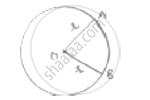# In a Circle of Radius 35 Cm, an Arc Subtends an Angle of 72° at the Centre. Find the Length of Arc and Area of Sector - Mathematics

In a circle of radius 35 cm, an arc subtends an angle of 72° at the centre. Find the length of arc and area of sector

#### Solution𝜃 = angle subtended at centre = 72°

Length of arc =theta/360^@× 2𝜋𝑟

=7/360× 2 ×22/7× 35

= 2 × 22 = 44 CMS

Area of sector =theta/360^@× pir^2

= 72/360×22/7× 35 × 35

= (35 × 22) = 770 cm2

Concept: Areas of Sector and Segment of a Circle
Is there an error in this question or solution?

#### APPEARS IN

RD Sharma Class 10 Maths
Chapter 13 Areas Related to Circles
Exercise 13.2 | Q 11 | Page 25